The spatially-adaptive solution of the azimuthally Fourier-decomposed equations of 3D time-harmonic linear elasticity on unstructured meshes

In this tutorial we re-visit the solution of the time-harmonic equations of 3D linear elasticity in cylindrical polar coordinates, using a Fourier decomposition of the solution in the azimuthal direction. The driver code is very similar to the one discussed in another tutorial – the main purpose of the current tutorial is to demonstrate the use of spatial adaptivity on unstructured meshes. Compared to the test case considered in the other tutorial we study a slightly less contrived test problem: the forced time-harmonic oscillations of a finite-length, hollow cylinder, loaded by a time-periodic pressure load on its inner surface.

 Acknowledgement: This implementation of the equations and the documentation were developed jointly with Robert Harter (Thales Underwater Systems Ltd) with financial support from a KTA Secondment grant from University of Manchester's EPSRC-funded Knowledge Transfer Account.

# The test problem

The figure below shows the problem considered in this tutorial: an annular elastic body that occupies the region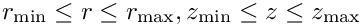is loaded by a time-harmonic pressure load acting on its inner surface (at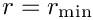). The upper and lower ends of the hollow cylinder (at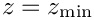and) are held at a fixed position.

Here is an animation of the resulting displacement field for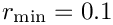and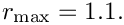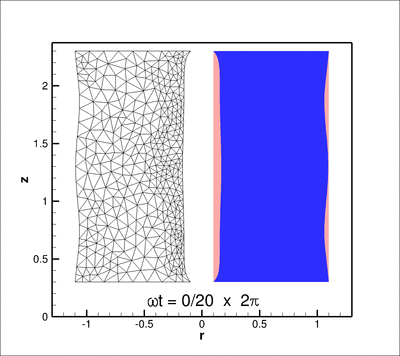Forced oscillations of a thick-walled, hollow cylinder, subject to a pressure load on its inner surface. The pink shape in the background shows the cylinder's undeformed shape (in a radial plane); the mesh plotted in the region r < 0 illustrates how spatial adaptivity refines the mesh in regions of sharp displacement gradients (near the loaded surface and the supports).

# The numerical solution

The driver code for this problem is very similar to the one discussed in another tutorial. Running sdiff on the two driver codes

demo_drivers/time_harmonic_fourier_decomposed_linear_elasticity/cylinder/cylinder.cc

and

shows you the differences, the most important of which are:

• The change of the forcing to a spatially constant pressure load on the inside boundary.
• The provision of the actions_before/after_adapt() functions and a helper function complete_problem_setup() which rebuilds the elements (by passing the problem parameters to the elements) following the unstructured mesh adaptation. (The need/rationale for such a function is discussed in another tutorial.)
• The mesh generation and the application of boundary conditions at the upper and lower boundaries of the hollow cylinder. .
All of this is reasonably straightforward and provides a powerful code that automatically adapts the mesh in regions of large displacement gradients. Have a look through the driver code and play with it.

# Code listing

Here's a listing of the complete driver code:

//LIC// ====================================================================
//LIC// This file forms part of oomph-lib, the object-oriented,
//LIC// multi-physics finite-element library, available
//LIC// at http://www.oomph-lib.org.
//LIC//
//LIC// Copyright (C) 2006-2022 Matthias Heil and Andrew Hazel
//LIC//
//LIC// This library is free software; you can redistribute it and/or
//LIC// modify it under the terms of the GNU Lesser General Public
//LIC// version 2.1 of the License, or (at your option) any later version.
//LIC//
//LIC// This library is distributed in the hope that it will be useful,
//LIC// but WITHOUT ANY WARRANTY; without even the implied warranty of
//LIC// MERCHANTABILITY or FITNESS FOR A PARTICULAR PURPOSE. See the GNU
//LIC// Lesser General Public License for more details.
//LIC//
//LIC// You should have received a copy of the GNU Lesser General Public
//LIC// License along with this library; if not, write to the Free Software
//LIC// Foundation, Inc., 51 Franklin Street, Fifth Floor, Boston, MA
//LIC// 02110-1301 USA.
//LIC//
//LIC// The authors may be contacted at oomph-lib@maths.man.ac.uk.
//LIC//
//LIC//====================================================================
// Driver
#include "generic.h"
#include "time_harmonic_fourier_decomposed_linear_elasticity.h"
// The mesh
#include "meshes/triangle_mesh.h"
using namespace std;
using namespace oomph;
//===start_of_namespace=================================================
/// Namespace for global parameters
//======================================================================
{
/// Define Poisson's ratio Nu
std::complex<double> Nu(0.3,0.0);
/// Define the non-dimensional Young's modulus
std::complex<double> E(1.0,0.0);
/// Define Fourier wavenumber
/// Define the non-dimensional square angular frequency of
/// time-harmonic motion
std::complex<double> Omega_sq (10.0,0.0);
/// Length of domain in r direction
double Lr = 1.0;
/// Length of domain in z-direction
double Lz = 2.0;
// Set up min & max (r,z) coordinates
double rmin = 0.1;
double zmin = 0.3;
double rmax = rmin+Lr;
double zmax = zmin+Lz;
/// Define the imaginary unit
const std::complex<double> I(0.0,1.0);
double P=1.0;
/// The traction function at r=rmin: (t_r, t_z, t_theta)
void boundary_traction(const Vector<double> &x,
const Vector<double> &n,
Vector<std::complex<double> > &result)
{
result = P;
// Axial traction
result = 0.0;
// Azimuthal traction
result = 0.0;
}
} // end_of_namespace
//===start_of_problem_class=============================================
/// Class to validate time harmonic linear elasticity (Fourier
/// decomposed)
//======================================================================
template<class ELEMENT>
{
public:
/// Constructor: Pass number of elements in r and z directions
/// and boundary locations
const unsigned &nr, const unsigned &nz,
const double &rmin, const double& rmax,
const double &zmin, const double& zmax);
/// Update before solve is empty
/// Update after solve is empty
/// Delete traction elements
/// Helper function to complete problem setup
/// Actions before adapt: Wipe the mesh of traction elements
{
// Kill the traction elements and wipe surface mesh
// Rebuild the Problem's global mesh from its various sub-meshes
rebuild_global_mesh();
}
/// Actions after adapt: Rebuild the mesh of traction elements
{
// Create traction elements from all elements that are
// Rebuild the Problem's global mesh from its various sub-meshes
rebuild_global_mesh();
// Complete problem setup
}
/// Doc the solution
void doc_solution(DocInfo& doc_info);
private:
/// Allocate traction elements on the bottom surface
/// Pointer to the bulk mesh
RefineableTriangleMesh<ELEMENT>* Bulk_mesh_pt;
#else
/// Pointer to the bulk mesh
Mesh* Bulk_mesh_pt;
#endif
/// Pointer to the mesh of traction elements
}; // end_of_problem_class
//===start_of_constructor=============================================
/// Problem constructor: Pass number of elements in coordinate
/// directions and size of domain.
//====================================================================
template<class ELEMENT>
(const unsigned &nr, const unsigned &nz,
const double &rmin, const double& rmax,
const double &zmin, const double& zmax)
{
// The boundary is bounded by four distinct boundaries, each
// represented by its own polyline
Vector<TriangleMeshCurveSection*> boundary_polyline_pt(4);
// Vertex coordinates on boundary
Vector<Vector<double> > bound_coords(2);
bound_coords.resize(2);
bound_coords.resize(2);
// Horizontal bottom boundary
bound_coords=rmin;
bound_coords=zmin;
bound_coords=rmax;
bound_coords=zmin;
// Build the boundary polyline
unsigned boundary_id=0;
boundary_polyline_pt=new TriangleMeshPolyLine(bound_coords,boundary_id);
// Vertical outer boundary
bound_coords=rmax;
bound_coords=zmin;
bound_coords=rmax;
bound_coords=zmax;
// Build the boundary polyline
boundary_id=1;
boundary_polyline_pt=new TriangleMeshPolyLine(bound_coords,boundary_id);
// Horizontal top boundary
bound_coords=rmax;
bound_coords=zmax;
bound_coords=rmin;
bound_coords=zmax;
// Build the boundary polyline
boundary_id=2;
boundary_polyline_pt=new TriangleMeshPolyLine(bound_coords,boundary_id);
// Vertical inner boundary
bound_coords=rmin;
bound_coords=zmax;
bound_coords=rmin;
bound_coords=zmin;
// Build the boundary polyline
boundary_id=3;
boundary_polyline_pt=new TriangleMeshPolyLine(bound_coords,boundary_id);
// Pointer to the closed curve that defines the outer boundary
TriangleMeshClosedCurve* closed_curve_pt=
new TriangleMeshPolygon(boundary_polyline_pt);
// Use the TriangleMeshParameters object for helping on the manage of the
// TriangleMesh parameters
TriangleMeshParameters triangle_mesh_parameters(closed_curve_pt);
// Specify the maximum area element
double uniform_element_area=0.2;
triangle_mesh_parameters.element_area() = uniform_element_area;
// Create the mesh
Bulk_mesh_pt=new RefineableTriangleMesh<ELEMENT>(triangle_mesh_parameters);
// Set error estimator
Bulk_mesh_pt->spatial_error_estimator_pt()=new Z2ErrorEstimator;
#else
//Now create the mesh
#endif
//Create the surface mesh of traction elements
Surface_mesh_pt=new Mesh;
assign_traction_elements();
// Complete problem setup
complete_problem_setup();
// Add the submeshes to the problem
// Now build the global mesh
build_global_mesh();
// Assign equation numbers
cout << assign_eqn_numbers() << " equations assigned" << std::endl;
} // end of constructor
//===start_of_complete_problem_setup=================================
/// Complete problem setup
//===================================================================
template<class ELEMENT>
{
// Set the boundary conditions for this problem: All nodes are
// free by default -- just pin & set the ones that have Dirichlet
// conditions here
// Pin displacements everywhere apart from boundaries 1 and 3
//-----------------------------------------------------------
for (unsigned ibound=0;ibound<3;ibound=ibound+2)
{
unsigned num_nod=Bulk_mesh_pt->nboundary_node(ibound);
for (unsigned inod=0;inod<num_nod;inod++)
{
// Get pointer to node
Node* nod_pt=Bulk_mesh_pt->boundary_node_pt(ibound,inod);
// Pinned in r, z and theta
nod_pt->pin(0);nod_pt->pin(1);nod_pt->pin(2);
nod_pt->pin(3);nod_pt->pin(4);nod_pt->pin(5);
// Set the displacements
nod_pt->set_value(0,0.0);
nod_pt->set_value(1,0.0);
nod_pt->set_value(2,0.0);
nod_pt->set_value(3,0.0);
nod_pt->set_value(4,0.0);
nod_pt->set_value(5,0.0);
}
}
// Complete the problem setup to make the elements fully functional
// Loop over the elements
unsigned n_el = Bulk_mesh_pt->nelement();
for(unsigned e=0;e<n_el;e++)
{
// Cast to a bulk element
ELEMENT *el_pt = dynamic_cast<ELEMENT*>(Bulk_mesh_pt->element_pt(e));
// Set the pointer to Poisson's ratio
el_pt->nu_pt() = &Global_Parameters::Nu;
// Set the pointer to Fourier wavenumber
el_pt->fourier_wavenumber_pt() = &Global_Parameters::Fourier_wavenumber;
// Set the pointer to non-dim Young's modulus
el_pt->youngs_modulus_pt() = &Global_Parameters::E;
// Set the pointer to square of the angular frequency
el_pt->omega_sq_pt() = &Global_Parameters::Omega_sq;
}// end loop over elements
// Loop over the traction elements
unsigned n_traction = Surface_mesh_pt->nelement();
for(unsigned e=0;e<n_traction;e++)
{
// Cast to a surface element
TimeHarmonicFourierDecomposedLinearElasticityTractionElement<ELEMENT>*
el_pt =
dynamic_cast<TimeHarmonicFourierDecomposedLinearElasticityTractionElement
<ELEMENT>* >(Surface_mesh_pt->element_pt(e));
// Set the applied traction
el_pt->traction_fct_pt() = &Global_Parameters::boundary_traction;
}// end loop over traction elements
}
//===start_of_traction===============================================
/// Make traction elements along the boundary r=rmin
//===================================================================
template<class ELEMENT>
{
unsigned bound, n_neigh;
// How many bulk elements are next to boundary 3
bound=3;
n_neigh = Bulk_mesh_pt->nboundary_element(bound);
// Now loop over bulk elements and create the face elements
for(unsigned n=0;n<n_neigh;n++)
{
// Create the face element
FiniteElement *traction_element_pt
= new TimeHarmonicFourierDecomposedLinearElasticityTractionElement<ELEMENT>
(Bulk_mesh_pt->boundary_element_pt(bound,n),
Bulk_mesh_pt->face_index_at_boundary(bound,n));
}
} // end of assign_traction_elements
//===start_of_delete_traction========================================
/// Delete traction elements
//===================================================================
template<class ELEMENT>
{
// How many surface elements are in the surface mesh
unsigned n_element = Surface_mesh_pt->nelement();
// Loop over the surface elements
for(unsigned e=0;e<n_element;e++)
{
// Kill surface element
delete Surface_mesh_pt->element_pt(e);
}
// Wipe the mesh
Surface_mesh_pt->flush_element_and_node_storage();
} // end of delete_traction_elements
//==start_of_doc_solution=================================================
/// Doc the solution
//========================================================================
template<class ELEMENT>
doc_solution(DocInfo& doc_info)
{
ofstream some_file;
char filename;
// Number of plot points
unsigned npts=5;
// Output solution
sprintf(filename,"%s/soln.dat",doc_info.directory().c_str());
some_file.open(filename);
Bulk_mesh_pt->output(some_file,npts);
some_file.close();
// Output norm of solution (to allow validation of solution even
// if triangle generates a slightly different mesh)
sprintf(filename,"%s/norm.dat",doc_info.directory().c_str());
some_file.open(filename);
double norm=0.0;
unsigned nel=Bulk_mesh_pt->nelement();
for (unsigned e=0;e<nel;e++)
{
double el_norm=0.0;
Bulk_mesh_pt->compute_norm(el_norm);
norm+=el_norm;
}
some_file << norm << std::endl;
} // end_of_doc_solution
//===start_of_main======================================================
/// Driver code
//======================================================================
int main(int argc, char* argv[])
{
// Number of elements in r-direction
unsigned nr=10;
// Number of elements in z-direction (for (approximately) square elements)
unsigned nz=unsigned(double(nr)*Global_Parameters::Lz/Global_Parameters::Lr);
// Set up doc info
DocInfo doc_info;
// Set output directory
doc_info.set_directory("RESLT");
// Set up problem
<ProjectableTimeHarmonicFourierDecomposedLinearElasticityElement
<TTimeHarmonicFourierDecomposedLinearElasticityElement<3> > >
// Solve
#else
// Set up problem
<QTimeHarmonicFourierDecomposedLinearElasticityElement<3> >
// Solve
problem.newton_solve();
#endif
// Output the solution
problem.doc_solution(doc_info);
} // end_of_main
Class to validate time harmonic linear elasticity (Fourier decomposed)
Definition: cylinder.cc:134
Actions before adapt: Wipe the mesh of traction elements.
FourierDecomposedTimeHarmonicLinearElasticityProblem(const unsigned &nr, const unsigned &nz, const double &rmin, const double &rmax, const double &zmin, const double &zmax)
Constructor: Pass number of elements in r and z directions and boundary locations.
Definition: cylinder.cc:174
Actions after adapt: Rebuild the mesh of traction elements.
Mesh * Surface_mesh_pt
Pointer to the mesh of traction elements.
Definition: cylinder.cc:163
void assign_traction_elements()
Allocate traction elements on the bottom surface.
Definition: cylinder.cc:289
Mesh * Bulk_mesh_pt
Pointer to the bulk mesh.
Definition: cylinder.cc:160
void actions_after_newton_solve()
Update after solve is empty.
Definition: cylinder.cc:149
void actions_before_newton_solve()
Update before solve is empty.
Definition: cylinder.cc:146
void complete_problem_setup()
Helper function to complete problem setup.
void doc_solution(DocInfo &doc_info)
Doc the solution.
Definition: cylinder.cc:318
int main(int argc, char *argv[])
Driver code.
Definition: cylinder.cc:360
Namespace for global parameters.
Definition: cylinder.cc:43
double Lz
Length of domain in z-direction.
Definition: cylinder.cc:65
const std::complex< double > I(0.0, 1.0)
Define the imaginary unit.
double Lr
Length of domain in r direction.
Definition: cylinder.cc:62
void boundary_traction(const Vector< double > &x, const Vector< double > &n, Vector< std::complex< double > > &result)
The traction function at r=rmin: (t_r, t_z, t_theta)
Definition: cylinder.cc:77
std::complex< double > Nu(0.3, 0.05)
Define Poisson's ratio Nu.
std::complex< double > Omega_sq(10.0, 5.0)
Define the non-dimensional square angular frequency of time-harmonic motion.
std::complex< double > E(1.0, 0.01)
Define the non-dimensional Young's modulus.
int Fourier_wavenumber
Define Fourier wavenumber.
Definition: cylinder.cc:55